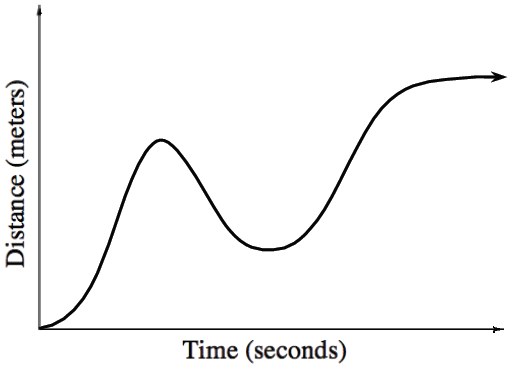### Home > APCALC > Chapter 3 > Lesson 3.3.3 > Problem3-115

3-115.

The graph at right shows the distance from a fixed point traveled by a toy car. Use the graph to sketch a velocity graph for the car.

Notice that shortly into the car's journey, it changed directions and started moving back towards its starting position. How will this be reflected on a velocity graph?

A steep negative slope on a distance graph will be plotted as a very low $y$-value on a velocity graph. A steep positive slope on a distance graph will be plotted as a very high $y$-value on a velocity graph. No slope on a distance graph will be plotted on the $x$-axis of the velocity graph.